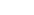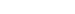# Qualitative Structural Analysis of Beams and FramesReading time: 1 minute

Qualitative structural analysis might not be familiar to structural engineers. The usual way of analysis of structures is numerical (quantitative) method in which the structural designers should determine values for dimensions and loads and compute bending moments and reactions. However, there is an important step before numerical structural analysis that is called preliminary analysis. In this stage dimensions of elements should be assumed before analysis is performed. Naturally, the detailed numerical analysis is to check values that have been employed in the preliminary analysis. Therefore, unless the structural elements sized correctly, the analysis has to be repeated many times regardless of using computer or hand calculation. This could be time consuming and costly and the designer should try to avoid this situation. By and large, it is considered that required knowledge and skills to carry out suitable and correct preliminary analysis comes from researching and studying techniques of numerical analysis, but this might not be the case. Qualitative structural analysis provides the necessary skills and knowledge to perform preliminary analysis. The foundation of these skills is to understand or recognize relations between loads and resulting structural behaviors. For example, in simple elements the basic skills are to recognize the relationships between loads, deflected shape and resulting reactions. Qualitative structural analysis is substantially different from quantitative method. Not only it does not have a specific solution procedure but also it depends on a number of diagrams. In qualitative analysis the procedure depends on the problem for example, in a specific problem starting solution from deflected shape could be suitable while for other problems beginning from bending moment diagram is effective to get the solution. Qualitative structural analysis method takes time and requires a lot of practices to utilize correctly and avoiding confusion.

## Conditions of Structural Behavior in Qualitative Structural Analysis

There are numbers of certain structural behaviors that structural analysis depends on to carry out qualitative structural analysis and some of them are clear and obvious while others might not be: 1. Types of support and their restraints: the fully fixed support provide two reactions and one moment, pinned support has two reactions and roller support provide one reaction that is vertical as shown in Table 1:

Table 1 Types of supports and their reactions2. Moment at the hinge is equal to zero. 3. Remember: moment is equal to force multiply by distance. 4. Draw bending moment diagram on the tension side of the beam to keep consistent answers. 5. For all loading cases, the structure remains in the elastic ranges of material behavior. Stress is proportional to strain and deflections are directly proportional to loads (first assumption of elastic analysis of structures). 6. Deflections resulting from loading do not lead to develop secondary bending moments. As shown in Figure 1 ABC is a cantilever that carries two loads W1 & W2. The W2 develop bending moment at BC that leads to a horizontal deflection at B, which is called. Secondary momentis neglected. (Second assumption of elastic analysis of structures).Figure 1: Cantilever member ABC with loads, deflection and bending moment diagram

7. In qualitative analysis, axial loads in members which create axial strains are neglected in bending moment determination. 8. Principle of superposition, which helps to simplify complex structure and examine loads differently, is considered to apply provided that the structure remains in elastic range. For example: Two span beams ABC is shown Figure 2 with deflected shape, reactions and bending moment diagram. Using principle of superposition, the indeterminate beam can be solved easily. Firstly, remove support C that lead to simply support and cantilever beam then draw the bending moment diagram as shown in left side of Figure 3 .Secondly, apply force Vc at C and draw BMD as shown in right side of Figure 3. These two diagrams provide the solution of the original two span beams. Similarly, deflections could be treated in the same manner.Figure 2 Beam ABC with deflected shape, reactions, and bending moment diagramFigure 3: Remove reaction at C (left side), remove W and recall reaction C right side, and solve both to get the original solution

9. There is no bending moment in the part of the structure that remains straight after loading, however it could move. 10. Bending moment diagrams will be straight lines if applied loads were point loads. 11. Bending moment diagrams will be curved if applied loads were distributed loads. 12. At simply supports, bending moment is equal to zero. 13. Bending moment diagrams cross elements at points of contra flexure. 14. Unbraced frames will not sway if it is symmetrical and symmetrically loaded. 15. Qualitative analysis is carried out for visible loads, therefore self weight is neglected. 16. Apart from at hinges, shapes of deflected elements are extremely smooth. 17. In frames, angles of rigid joints should maintain the original angles after loadings as shown in Figure 4.Figure 4: Angle of rigid joint frame keep the same angle after loading

18. In frames, rigid joints might close or open only as shown in .Figure 5: Open and close rigid joints after loading with bending moment diagrams

19. There is only one moment value in the rigid joints that connects two elements. 20. Shear forces become axial force and axial force become shear force in the alternate members at the right angle rigid join. sum(fx)  = 0 , sum(fy) = 0 can be employed to check this statement. See Figure 6Figure 6: Right angle rigid join in which shear force become axial and axial force become shear force in the alternate member.

However, this is not the case in the oblique angle join as shown in Figure 7Figure 7: Oblique angle joint

21. In rigid joints in which there are more than two members, the joint must be in equilibrium. As shown in Figure 8, in the following joint MA = MB+MCFigure 8: Equilibrium rigid joint in which three members are meet.

### A complete solution of qualitative structural analysis is composed of:

• Deflected shape
• Reactions
• Bending moment diagrams